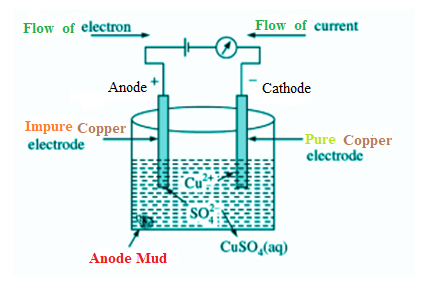QuestionAnswers

# In electro-refining of copper, some gold is deposited as:(A) Cathode mud(B) Electrolyte(C) Anode mud(D) None of theseHint: The Refining itself means to remove impurities or undesirable substances from a substance. So, electro-refining is a process to remove impurities using electricity. Basically, it's a process of refining in metals using electrolysis.

Here we will take an example of electrolytic copper refining to understand the process more clearly. Copper is typically mined from its coal referred to as copper. It is about 98 to 99 percent pure. However, the electro-refining process can easily make it 99.95% pure which makes it a good product to be used in electrical components.

A block of impure copper is taken as an anode or positive electrode. Copper sulphate which is acidified with vitriol is employed as a graphite-coated electrolyte alongside pure copper tubes, as a cathode or negative electrode. In this phase of electrolysis copper sulphate divides into a positive ion of copper ($C{{u}^{2+}}$) and a negative ion of sulphate ($S{{O}_{4}}^{-}$). The positive copper ion ($C{{u}^{2+}}$) or cations travel towards the negative electrode made from pure copper where it absorbs the electrons from the cathode. Cu atoms are deposited on the cathode’s graphite layer.

The cathode is coated with graphite in the process of electrolytic metal processing or merely electro grinding so that the concentrated material can be easily removed. This is one of the most growing electrolysis procedures.

Electrolytic Refining of CopperHowever, anode’s metallic impurities are also mixed with $S{{O}_{4}}$, forming metallic sulphate in the electrolyte solution and dissolving. In this above process, some impurities get dissolved in the solution while some deposits as anode mud below the anode electrode. Impurities which dissolve within the solution are Fe, Ni, Zn while impurities like Au, Ag and Pt deposits as anode mud below the anode electrode.
So, we can say that gold (Au) is deposited as Anode mud below the anode electrode.

Therefore, among the options if we check then option (C)Anode mud is the correct answer of the question.

Note:
- ${{H}^{+}}$from the electrolyte isn't reduced to ${{H}_{2}}$ (g) at the cathode.
-$C{{u}^{2+}}$lies below ${{H}^{+}}$within the table of ordinary reduction potentials.
-$C{{u}^{2+}}$is a stronger oxidant than ${{H}^{+}}$.
-$C{{u}^{2+}}$is more easily reduced than ${{H}^{+}}$.
-Keep in mind the impurities like Fe, Ni, Zn get dissolved within the solution but impurities like Au, Ag and Pt are deposited as anode mud below the anode.

View Notes
Organic Chemistry - Some Basic Principles and TechniquesDifference Between Galvanic Cells and Electrolytic CellsNCERT Book for Class 11 Chemistry PDF in HindiSome Natural PhenomenaWhat is the full form of phd?Table of 11 - Multiplication Table of 11NCERT Book Class 11 Chemistry PDFUses of Alloys in Daily LifeClassification of Elements and Periodicity in PropertiesWhat is Set, Types of Sets and Their Symbols?Important Questions for CBSE Class 11 Chemistry Chapter 1 - Some Basic Concepts of ChemistryNCERT Books Free Download for Class 11 Chemistry Chapter 1 - Some Basic Concepts of ChemistryNCERT Books Free Download for Class 11 Chemistry Chapter 12 - Organic Chemistry - Some Basic Principles and TechniquesImportant Questions for CBSE Class 11 Chemistry Chapter 12 - Organic Chemistry - Some Basic Principles and TechniquesNCERT Books Free Download for Class 11 Chemistry Chapter 3 - Classification of Elements and Periodicity in PropertiesImportant Questions for CBSE Class 11 Chemistry Chapter 3 - Classification of Elements and Periodicity in PropertiesImportant Questions for CBSE Class 11 Chemistry Chapter 5 - States of MatterImportant Questions for CBSE Class 11 Chemistry Chapter 2 - Structure of AtomNCERT Books Free Download for Class 11 Chemistry Chapter 5 - States of MatterNCERT Books Free Download for Class 11 Chemistry Chapter 2 - Structure of AtomChemistry Question Paper for CBSE Class 12CBSE Class 12 Chemistry Question Paper 2020CBSE Class 12 Chemistry Question Paper 2019 - Free PDFChemistry Question Paper for CBSE Class 12 - 2013Chemistry Question Paper for CBSE Class 12 - 2015CBSE Class 12 Chemistry Question Paper 2018 - Free PDFCBSE Class 12 Chemistry Question Paper 2017 - Free PDFPrevious Year Question Paper for CBSE Class 12 Chemistry - 2014Chemistry Question Paper for CBSE Class 12 - 2016 Set 1 EChemistry Question Paper for CBSE Class 12 - 2016 Set 1 SNCERT Solutions for Class 11 Chemistry Chapter 1 Some Basic Concepts of Chemistry in HindiNCERT Solutions Class 11 Chemistry Chapter 1NCERT Exemplar for Class 11 Chemistry Chapter 1 - Some Basic Concepts of Chemistry (Book Solutions)NCERT Solutions for Class 11 Chemistry Chapter 12 Organic Chemistry Some Basic Principles and Techniques In HindiNCERT Solutions for Class 11 Chemistry Chapter 12NCERT Solutions for Class 11 Chemistry Chapter 2 Structure of Atom In HindiNCERT Solutions for Class 11 Chemistry Chapter 5 States of Matter In HindiNCERT Solutions for Class 11 Chemistry Chapter 3 Classification of Elements and Periodicity in Properties In HindiNCERT Solutions for Class 11 Chemistry in HindiNCERT Solutions for Class 11 Chemistry Chapter 14 Environmental Chemistry In Hindi